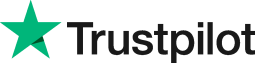How to calculate the difference between two times in Excel?
• Home
• Courses
• Promotions
• Schedule
• Formats
• Our Clients

Forum home » Topics » How to calculate the difference between two times in Excel?

# How to calculate the difference between two times in Excel?

Excel allows you to carry out calculations using date and time data.

The commercial benefit to this feature is that you can set up spreadsheets to calculate dates and times that effect formulas and functions automatically. for example you could use this feature to manage your bad debt based on the date fomulas =today() or =now() and have you payments linked to these function using a formula.

When using time in a cell it is best practice to format the cell first using a Time Format, and then entering the time you want e.g. 08:30. Let's say you want to subtract one time value from another, first enter a value into a cell, say A1 and the second value into cell B1. Select cell C1 and enter the following formula:

=B1-A1 If A1 has 10:30 and B1 has 14:00 this formula would calculate the difference between the two times and give the result as 3:30

Please be aware that Excel does not give negative time results. These will be shown as ################ and to get around this you must use the options to change the calculations to use the 1904 date system.

# Related forum posts:

## Excel - time calculations

Hi there, I am trying to replicate a timesheet that we used in a previous role where the formula could calculate hours due/owed by the employee. I have managed to get the formula to work as long as the employee does their hours or over time but it will not show a negative result in the event that an employee does not do enough for that day. At present the calculation is relatively simple with a cell which adds up the finish time minus the start time and time taken for lunch (hours actual

# Other posts you might be interested in:

## Calculating Dates

Can I calculate on dates prior to 1900?

## Excel

I want to calculate no of working days in year by using hire and leaving dates.

## Dates

How do you work with two separate dates? For example find number of days between two dates?

# Related articles

## Stay In The Loop With VBA

What is the difference between a recorded macro in Microsoft Excel and a VBA written procedure? The answer-None, as all Excel is doing when you record a macro is translate each keystroke or mouse click to VBA.

## Using Dates Within Excel

 Welcome. Please choose your application (eg. Excel) and then post your question. Our Microsoft Qualified trainers will then respond within 24 hours (working days). Frequently Asked Questions What does 'Resolved' mean? Any suggestions, questions or comments? Please post in the Improve the forum thread.TrustScore 4.9 / 5       1244 reviews